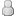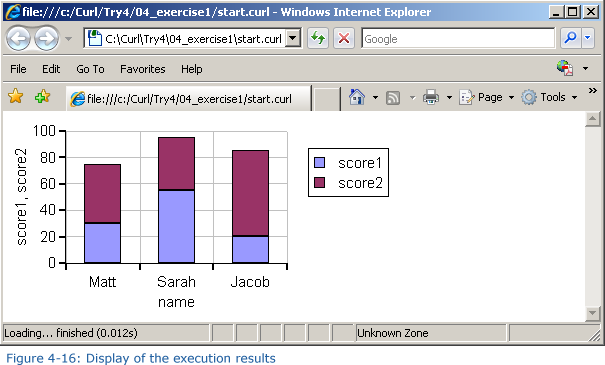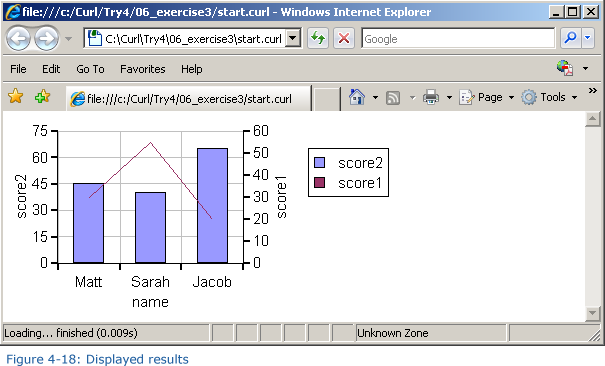• 504 Vote(s) - 2.79 Average
• 1
• 2
• 3
• 4
• 5Page 8: Extra Practice
06-20-2011, 01:10 PM, (This post was last modified: 06-23-2011, 04:20 PM by ashimo.)ashimoModeratorReputation: 3 Posts: 99 Joined: Jun 2011
Page 8: Extra Practice
Extra Practice

Let’s use options to change the format and colors of a graph.

Practice Problem 1: Basics

Define data like that shown below, and then display a cumulative bar graph for the score1 and score2 data fields. For the x-axis label, use the name field.

Code:
```let data:RecordSet = {RecordSet                          {RecordFields                              {RecordField name, domain = String},                              {RecordField score1, domain = int},                              {RecordField score2, domain = int}                          },                          {RecordData name = Matt, score1 = 30, score2 = 45},                          {RecordData name = Sarah, score1 = 55, score2 = 40},                          {RecordData name = Jacob, score1 = 20, score2 = 65}                      }```Hint: To display the cumulative graph, use stacking-mode = ChartStackingMode.stacked with BarLayer.

Solution

Solution Program: c:\Curl\Try4\04_exercise1\start.curl

Code:
```{import * from CURL.GUI.CHARTS} {value     let data:RecordSet =         {RecordSet             {RecordFields                 {RecordField name, domain = String},                 {RecordField score1, domain = int},                 {RecordField score2, domain = int}             },             {RecordData name = Matt, score1 = 30, score2 = 45},             {RecordData name = Sarah, score1 = 55, score2 = 40},             {RecordData name = Jacob, score1 = 20, score2 = 65}         }          let chart:LayeredChart = {LayeredChart                                  height = 5cm,                                  width = 10cm,                                  {BarLayer                                      stacking-mode = ChartStackingMode.stacked,                                      x-axis-data = {ChartDataSeries data, name},                                      {ChartDataSeries data, score1},                                      {ChartDataSeries data, score2}                                  }                              }     chart }```

Practice Problem 2: Basics

Display the data used with Practice Problem 1 as a bar graph. In this problem, reverse the directions of the x- and y-axes and then display the graph.Hint: To change the orientation of the graph, use flipped? = true with LayeredChart.

Solution

Solution Program: c:\Curl\Try4\05_exercise2\start.curl

Code:
```{import * from CURL.GUI.CHARTS} {value     let data:RecordSet =         {RecordSet             {RecordFields                 {RecordField name, domain = String},                 {RecordField score1, domain = int},                 {RecordField score2, domain = int}             },             {RecordData name = Matt, score1 = 30, score2 = 45},             {RecordData name = Sarah, score1 = 55, score2 = 40},             {RecordData name = Jacob, score1 = 20, score2 = 65}`         }          let chart:LayeredChart = {LayeredChart                                  height = 5cm,                                  width = 10cm,                                  flipped? = true,                                  {BarLayer                                      x-axis-data = {ChartDataSeries data, name},                                      {ChartDataSeries data, score1},                                      {ChartDataSeries data, score2}                                  }                              }     chart }```

Practice Problem 3: Basics

Using the same data as that used in Practice Problem 1, display the score1 data as a bar graph, and the score2 data as a line graph, as shown in the figure below:Hint: LayeredChart can have multiple ChartLayers.

Solution

Solution Program: c:\Curl\Try4\06_exercise3\start.curl

Code:
```{import * from CURL.GUI.CHARTS} {value     let data:RecordSet =         {RecordSet             {RecordFields                 {RecordField name, domain = String},                 {RecordField score1, domain = int},                 {RecordField score2, domain = int}             },             {RecordData name = Matt, score1 = 30, score2 = 45},             {RecordData name = Sarah, score1 = 55, score2 = 40},             {RecordData name = Jacob, score1 = 20, score2 = 65}         }          let chart:LayeredChart = {LayeredChart                                  height = 5cm,                                  width = 10cm,                                  {BarLayer                                      x-axis-data = {ChartDataSeries data, name},                                      {ChartDataSeries data, score2}                                  },                                  {LineLayer                                      {ChartDataSeries data, score1}                                  }                              }          chart }```
 « Next Oldest | Next Newest »

 Possibly Related Threads... Thread Author Replies Views Last Post Page 5: Application 2: Specifying the Graph Colors ashimo 1 5,739 11-19-2016, 01:59 PM Last Post: milijacob Page 7: Summary ashimo 0 3,282 06-20-2011, 01:09 PM Last Post: ashimo Page 6: Structure of a Program that Specifies the Graph Colors ashimo 0 3,428 06-20-2011, 01:08 PM Last Post: ashimo Page 4: Structure of a Program that Specifies the Graph Axes ashimo 0 3,496 06-20-2011, 01:06 PM Last Post: ashimo Page 3: Application 1: Specifying the Graph Axes ashimo 0 3,417 06-20-2011, 01:05 PM Last Post: ashimo Page 2: Structure of a Program that Displays Data as a Graph ashimo 0 3,413 06-20-2011, 01:03 PM Last Post: ashimo Page 1: Displaying Data as a Graph ashimo 0 3,160 06-20-2011, 11:59 AM Last Post: ashimo
Forum Jump: